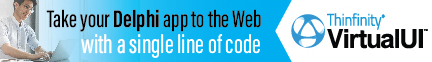DelphiBasicsArcSinFunction The Arc Sine of a number, returned in radians Math unit
 function ArcSin ( const Number : Extended ) : Extended;
Description
The ArcSin function is a mathematical function giving the value in radians of the Arc Sine of Number.

Notes
The System unit supports only the following angular functions:

ArcTan
Sin
Cos

Related commands
 ArcCos The Arc Cosine of a number, returned in radians ArcTan The Arc Tangent of a number, returned in radians Cos The Cosine of a number Sin The Sine of a number Tan The Tangent of a numberDownload this web site as a Windows program.